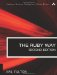# Section 7.17. Computing the Difference in Two DateTime Values

### 7.16. Adding Intervals to Date/Time Values

We can obtain a new time by adding an interval to a specified time. The number is interpreted as a number of seconds.

`t0 = Time.now t1 = t0 + 60         # Exactly one minute past t0 t2 = t0 + 3600       # Exactly one hour past t0 t3 = t0 + 86400      # Exactly one day past t0`

The function dhms2sec (defined in section 7.6 "Converting Between Seconds and Larger Units" earlier in the chapter) might be helpful here. Recall that the hours, minutes, and seconds all default to 0.

`t4 = t0 + dhms2sec(5,10)       # Ahead 5 days, 10 hours t5 = t0 + dhms2sec(22,18,15)   # Ahead 22 days, 18 hrs, 15 min t6 = t0 - dhms2sec(7)          # Exactly one week ago`

Don't forget that we can move backward in time by subtracting. This is shown in the calculation of t6 in the preceding code example.The Ruby Way, Second Edition: Solutions and Techniques in Ruby Programming (2nd Edition)
ISBN: 0672328844
EAN: 2147483647
Year: 2004
Pages: 269
Authors: Hal Fulton

Similar book on Amazon